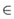# Reciprocal Function

This is the Reciprocal Function:

f(x) = 1/x

This is its graph:f(x) = 1/x

It is a Hyperbola.

It is an odd function.

Its Domain is the Real Numbers, except 0, because 1/0 is undefined.

Using set-builder notation:

Its Domain is {x| x ≠ 0}

Its Range is also {x| x ≠ 0}

## As an Exponent

The Reciprocal Function can also be written as an exponent:

f(x) = x-1

Plot the graph here